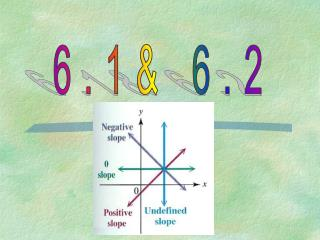DownloadDownload Presentation6.1& 6.2

# 6.1& 6.2

Télécharger la présentation## 6.1& 6.2

- - - - - - - - - - - - - - - - - - - - - - - - - - - E N D - - - - - - - - - - - - - - - - - - - - - - - - - - -
##### Presentation Transcript

1. 6.1& 6.2

2. SLOPE Slope is the ratio of the change of the vertical rise to the change in the horizontal run between any two points on a line. Usually referred to as the rise over run. Use a slope triangle between two points to determine rise and run. Rise is 10 because we went up Run is -6 because we went to the left

3. FORMULA FOR FINDING SLOPE ON A LINE The formula is used when you know two points of a line. Example: Find the slope of the line that passes through the points (3, 0) and (9,10)

4. Slope = 3/1 y-intercept Graphing using Slope and y-Intercept Example:Sketch the graph of f (x) = 3x – 1 y-axis x-axis

5. Slope m = -4 = -4 1 y-intercept (0, 1) Graph using slope-intercept form y = -4x + 1:

6. Slope m = 3 4 y-intercept (0, -2) Graph using slope-intercept form 3x - 4y = 8 y = 3x - 2 4

7. Review of Formulas Formula for Slope Standard Form *where A>0 and A, B, C are integers Slope-intercept Form

8. Parallel Lines • **Parallel lines have the same slopes. • Find the slope of the original line. • Use that slope to graph your new line and to write the equation of your new line. • Equations of a line passes through P(x1,y2) and has slope m is: • y –y1 = m(x-x1)

9. Graph a line parallel to the given line and through point (0, -1): Slope = 3 5

10. Write the equation of a line parallel to 2x – 4y = 8 and containing (-2, 4): – 4y = - 2x + 8 y = 1x - 2 2 Slope = 1 2 y - 4 = 1(x + 2) 2 y= 1x -3 2

11. Perpendicular Lines • **Perpendicular lines have the • opposite reciprocal slopes. • Find the slope of the original line. • Change the sign and invert the • numerator and denominator • of the slope. • Use that slope to graph your new • line and to write the equation • of your new line.

12. Graph a line perpendicular to the given line and through point (1, 0): Slope =-3 4 Perpendicular Slope= 4 3

13. Write the equation of a line perpendicular to 3x – 4y = 8 and containing (-3, 4): -4y = -3x + 8 Slope= 3 4 y - 4 = -4(x + 3) 3 Perpendicular Slope = -4 3 y = -4x 3

14. Write the equation of a line perpendicular to y = -2x + 3and containing (2, 8): Original Slope= -2 y = x + b 7 = 2 + b Perpendicular Slope = 1 2 8 = + b 16 = 2 + 2b 14 = 2b 7 = b y = x + 7

15. p. 340 #6ac, 9ac, 13a(i) (iii), 15ab, 17abcd, 18a(i) p. 349/350 #3ac,4ac,5ac,9ac,12,13